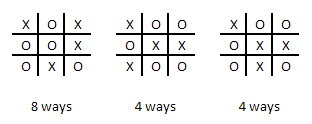# Tic Tac Toe

## Introduction

Tic Tac Toe is a casino game based on the actual game by the same name. A reader told me he saw it at Coushatta Casino Resort in Kinder, LA. There are three bets available: X, O, and a tie. Cards marked X or O are drawn randomly on a tic tac toe board to determine the winning outcome.

## Rules

1. The game utilizes four 52-card decks. Each deck consists of 26 cards marked X and 26 cards marked O.
2. Cards are drawn from the shoe and placed on the 9 squares of the tic tac toe board. Cards are placed until there is a winner (X or O with three in a row), or each square on the board is covered, whichever happens first.
3. A winning bet on X or O shall pay even money. In the event of a tie, bets on X or O shall lose half.
4. A winning bet on a tie shall pay 14 to 1.

## Analysis

The following table shows the probability of 0 to 9 X cards, and the probability of a tie given that number of Xs. The first column is the number of Xs out of 9. The second column is the probability of the given number of Xs on the card. The third column is the conditional probability of a tie, given the number of Xs for that row. The fourth column is the product of the second and third column. The lower right cell shows the probability of a tie is 6.3735%.

### Tie Probability in Tic Tac Toe — Four Decks

Number Xs Probability Conditional Probability of a Tie Product
0 0.001631 0 0
1 0.015898 0 0
2 0.067526 0 0
3 0.163991 0 0
4 0.250955 0.126984 0.031867
5 0.250955 0.126984 0.031867
6 0.163991 0 0
7 0.067526 0 0
8 0.015898 0 0
9 0.001631 0 0
Total 1.000000   0.063735

If there are 4 Xs and 5 Os, then there are combin(9,4)=126 ways to place the Xs and Os on the board. Of those 126, 16 result in a tie. The image below shows the three tie patterns, and the number of ways each can be rotated or reflected. So the probability of a tie, given 4 or 5 Xs, is 16/126 = 12.70%.Once it can be accepted that the probability of a tie is 6.3735%, the rest is easy. The probability that there is not a tie is 100%-6.3735% = 93.6265%. The probability of X or O winning is each half of that, or 46.8133%.

The following table shows the probability and contribution to the return of each outcome of the X and O bets. The lower right cell shows a house edge of 3.19%.

### X/O Bet Return — Four Decks

Event Probability Pays Return
Win 0.468133 1 0.468133
Tie 0.063735 -0.5 -0.031867
Loss 0.468133 -1 -0.468133
Total 1.000000   -0.031867

The following table shows the probability and contribution to the return of each outcome of the Tie bet. The lower right cell shows a house edge of 4.40%.

### Tie Bet Return — Four Decks

Event Probability Pays Return
Win 0.063735 14 0.892286
Loss 0.936265 -1 -0.936265
Total 1.000000   -0.043980

The final table shows the house edge of each bet for 4 to 8 decks, assuming no other rule changes.

Decks X/O Tie
4 3.19% 4.40%
5 3.17% 4.78%
6 3.17% 5.03%
7 3.16% 5.20%
8 3.16% 5.34%